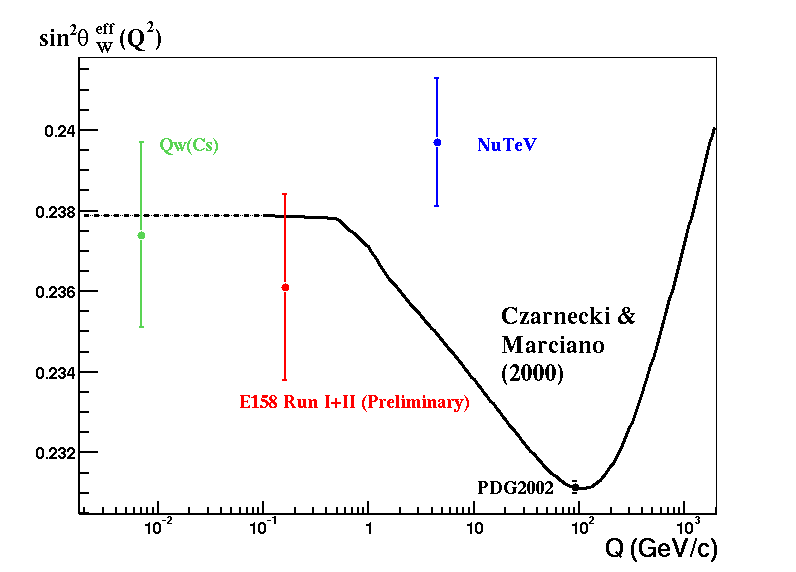Beyond Theta13

People:
• J. A. Formaggio
• M. H. Shaevitz
• V. Paolone

Topics:

Measuring the Electroweak ParametersThe high flux of neutrinos coming from nuclear reactors are currently being studied to measure the final oscillation parameter (q13) to high precision.  The settings for such a measurement can also be used to perform other high precision measurements involving the standard model.  Such precision tests may lead to discoveries of new physics.

One of the measurements that can be made at neutrino reactor experiments is a precise determination of the weak mixing angle, sin2(qW).  Such a measurement is motivated by the recent NuTeV result, whose 0.75% precision measurement shows a 3 s
igma deviation from what is predicted in the Standard Model.

The NuTeV measurement may be indicative of new physics beyond the standard model at low Q2.  As the measurement was made using high energy neutrinos, the best way to test for new physics is to again measure the weak mixing parameter with neutrinos at a similar
Q2 region.

Reactor experiments provide an ideal setting for measuring the weak mixing angle by studying neutrino-electron scattering.  Such a process is mediated entirely by the weak force and does not suffer from any corrections due to the strong force.  Reactor neutrino experiments can hope to attain a precision measurement of order ~1%.  Such precision is comparable to those achieved by Moller scattering and neutrino nuclear scattering.

The NuTeV Neutrino Experiment

The NuTeV Mixing Angle Measurement, by G. P. Zeller et al.

A Proposal to Measure the Weak Mixing Angle Using Reactor Neutrinos, by J. Conrad, M. Shaevitz, and J. Link

A Review of Electroweak Tests of the Standard Model

A Search for Light and Heavy Sterile NeutrinosA number of theories beyond the standard model predict the existence of "sterile" neutrinos; that is, neutrinos that do not couple directly to the W or Z boson.  Their existence provides a natural theoretical framework to understand the mechanism behind neutrino mass.   Sterile neutrinos can be extremely light (<1 eV) or relatively heavy (~MeV).  If they are light, they would help explain the LSND oscillation measurement.  If, on the other hands, they are heavy they may prove to be the missing link in understanding the formation of heavy elements in the universe.

Reactor neutrino experiments can be used to search for both light and heavy sterile neutrinos.  For example, if heavy sterile neutrinos couple to ordinary electron neutrinos, one can look for signatures of neutrino decay.  Such searches could be complementary to similar decay searches performed with solar neutrino experiments.

Decay of Reaction Neutrinos  by P. Vogel

New Experimental Limits on Heavy Neutrino Mixing from the Borexino CTF  the Borexino Collaboration

A Combined Analysis of Short Baseline Neutrino Experiments in the (3+1) and (3+2) Sterile Neutrino Hypothesis  by M. Sorel, M. Shaevitz and J. Conrad

Searching for Exotic Particles using the NuTeV Neutrino Beam  by J. Formaggio

Measuring the Neutrino Magnetic Moment

The neutrino magnetic moment, as predicted by the Standard Model with massive neutrinos arises from virtual loops of the W and, as such, is very, very small.  As a result, any measurement of a non-zero neutrino magnetic moment would be a clear sign of physics beyond the Standard Model.  the same techniques used for measuring the electroweak parameters can also be used to set limits on the neutrino magnetic moment.  Sensitivities to such physics is currently being explored.

Code to simulate radioactive backgrounds from beta-gamma decays  by J. Formaggio

Code to simulate muon and neutron flux at given depth  by J. Formaggio

Document on Fiducial Volume Studies by J. Conrad

Meeting Notes